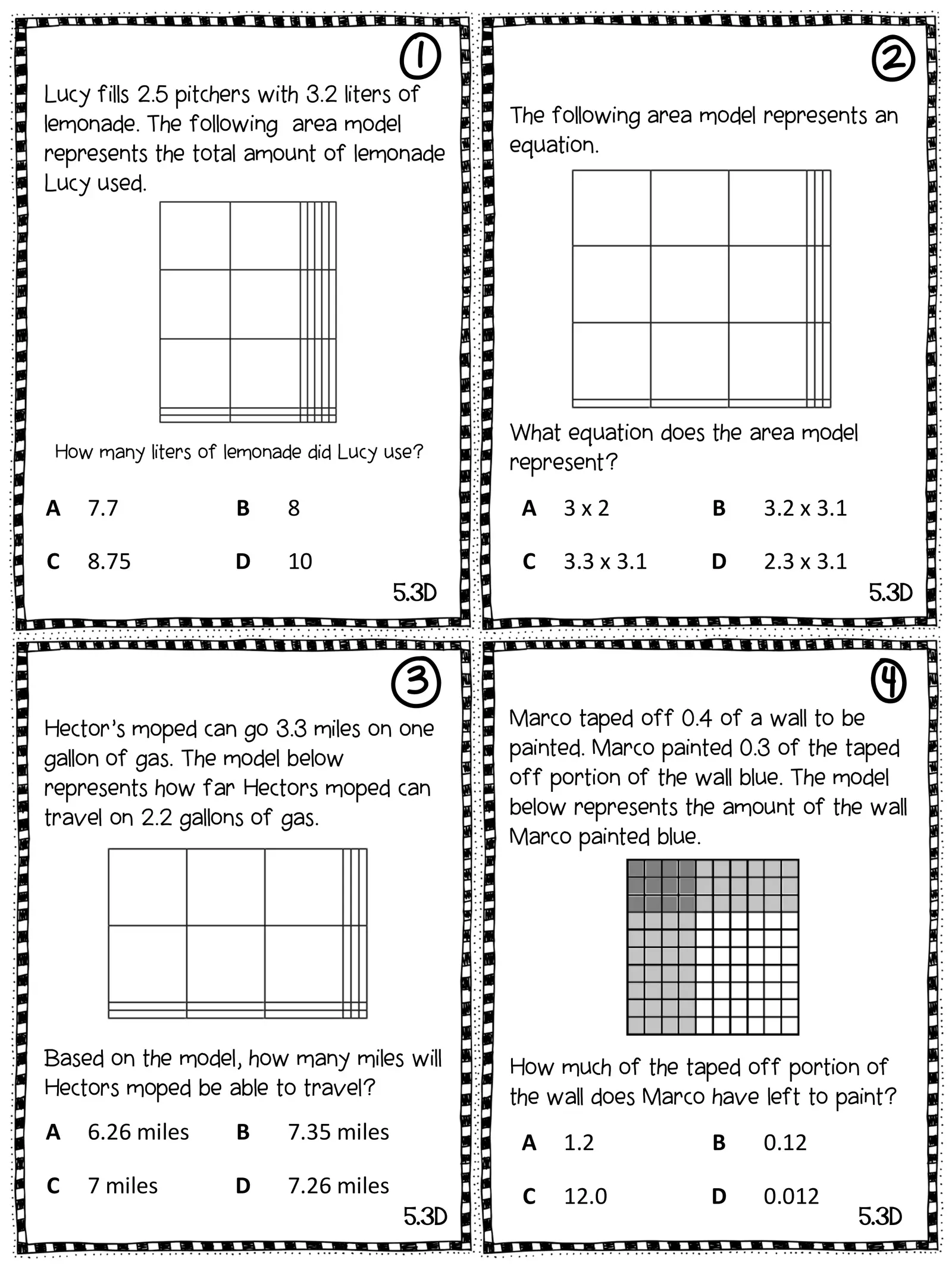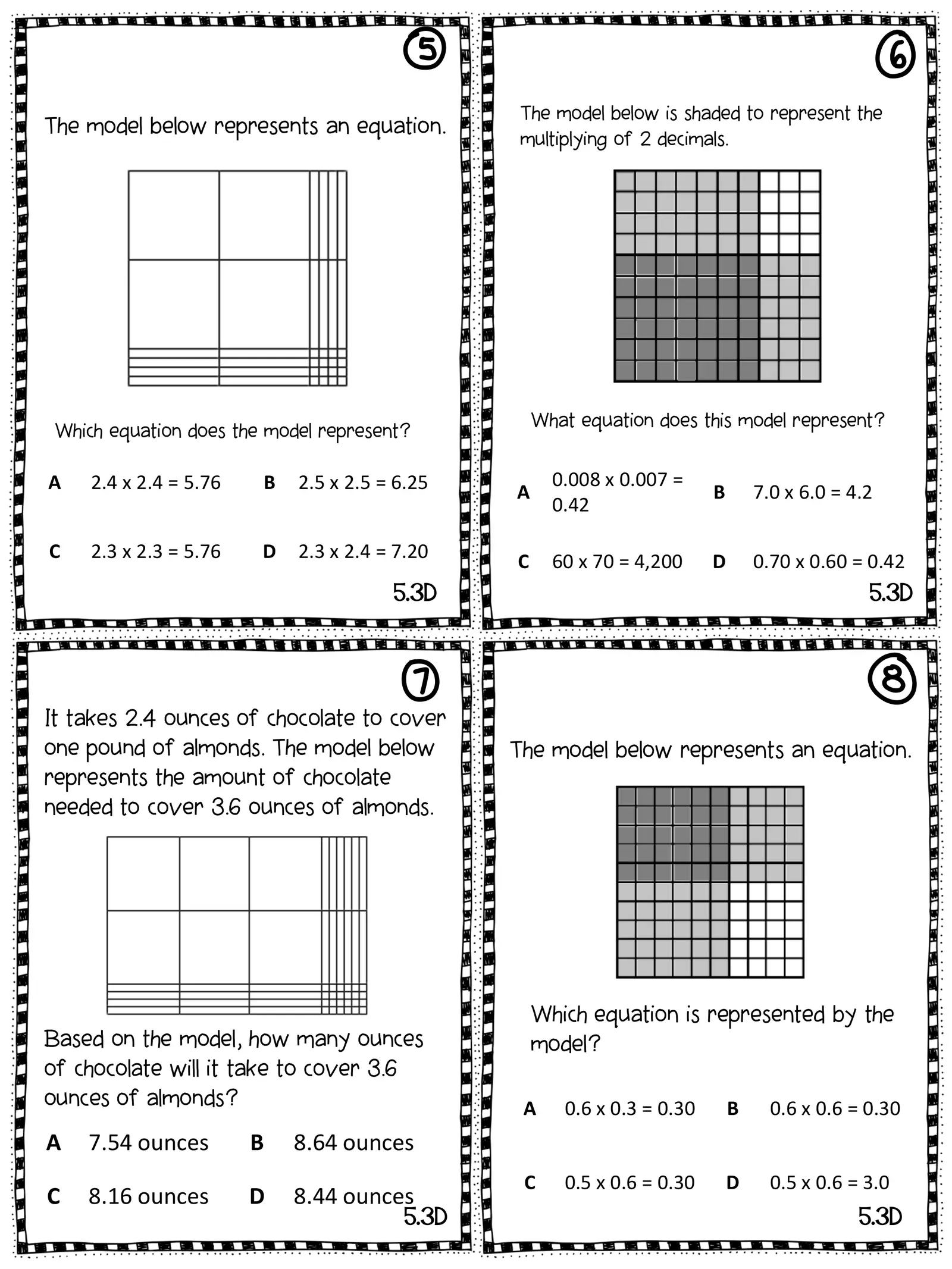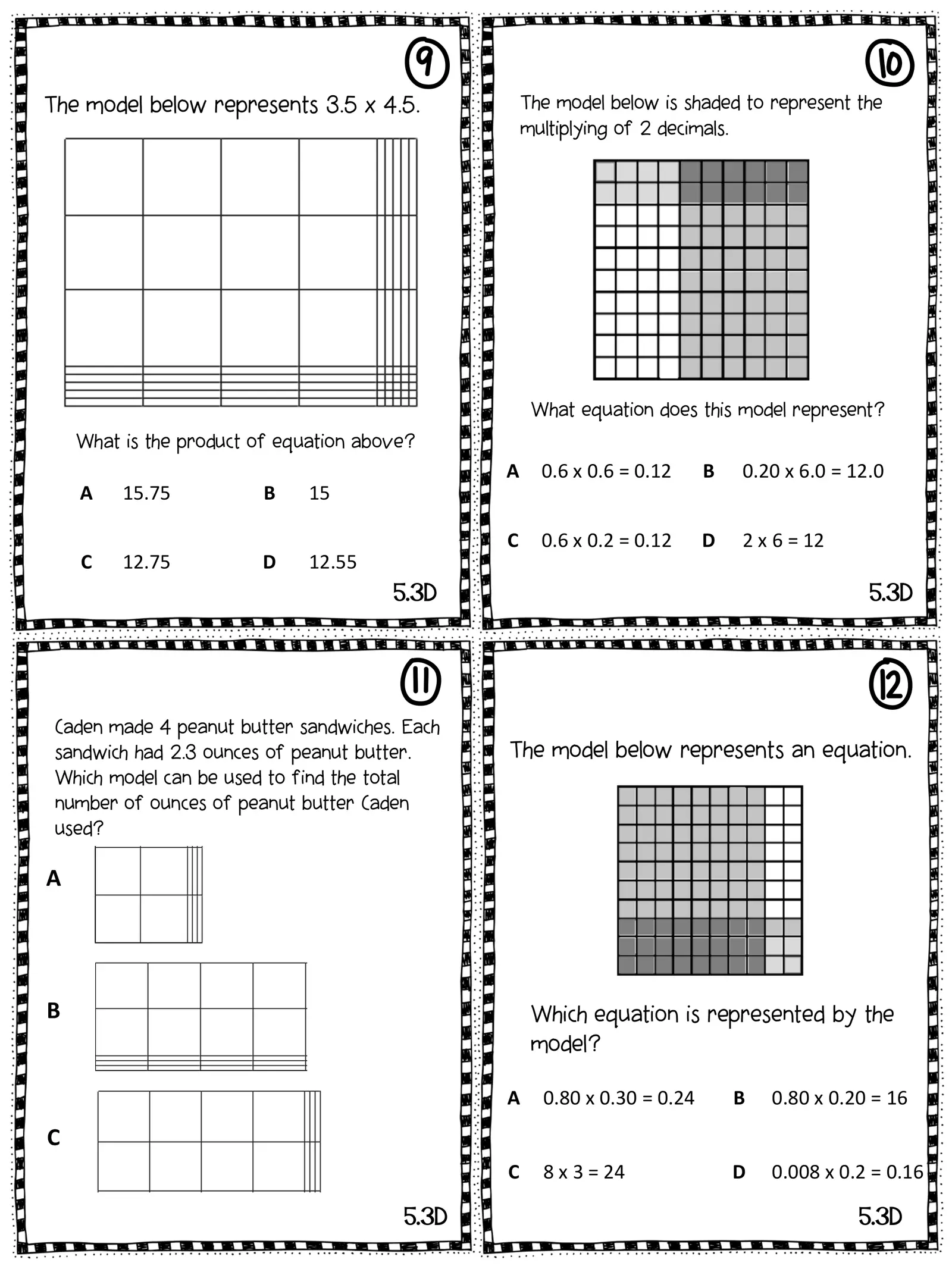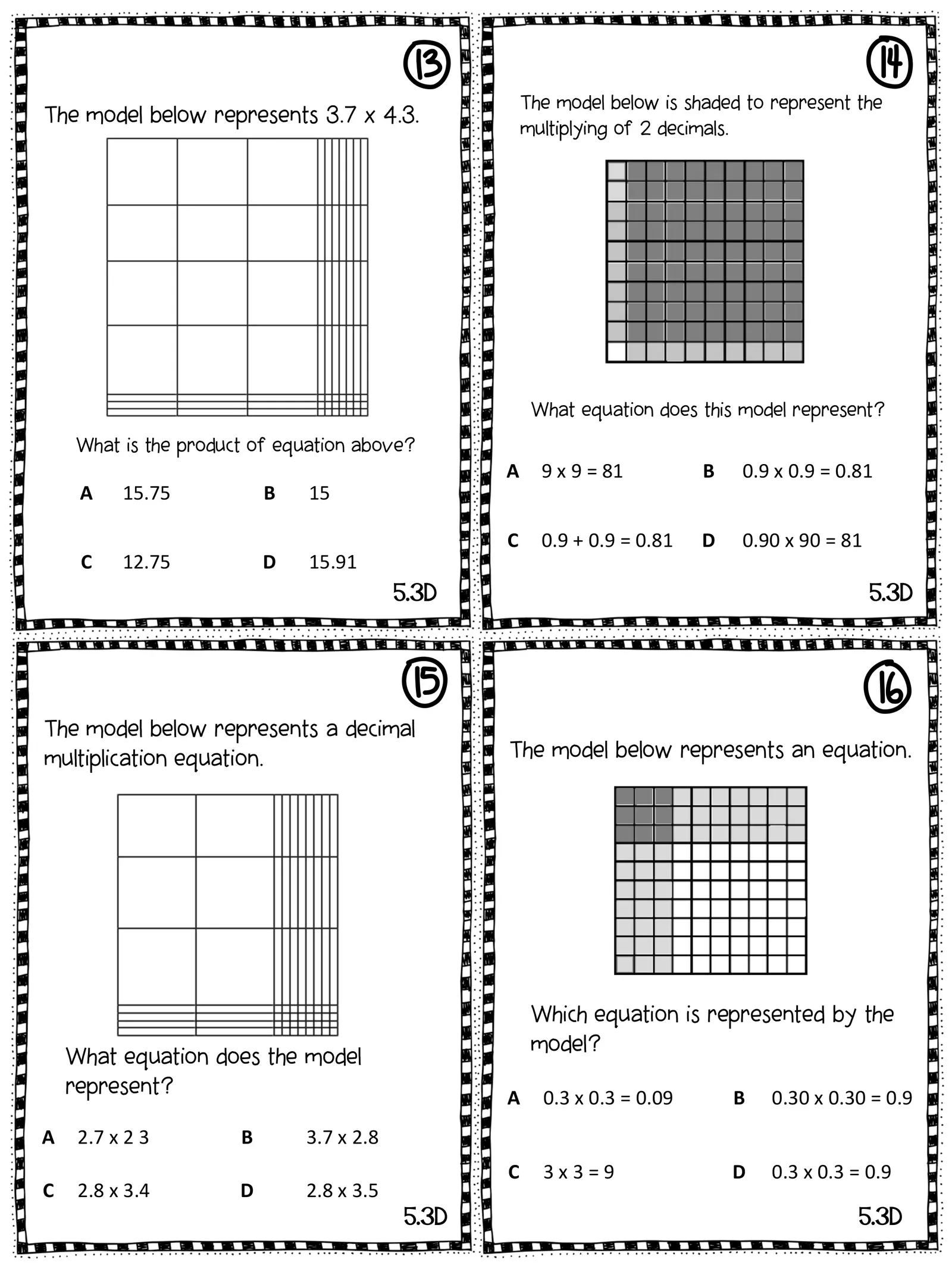Created
Best for live in-class or video conferencing lessonsStart teacher-led lessonE F 1 The model below is shaded to represent the The model below represents an equation. multiplying of 2 decimals. What equation does this model represent? Which equation does the model represent? A 2.4 x 2.4 = 5.76 B 2.5 x 2.5 = 6.25 0.008 x 0.007 = A B 7.0 x 6.0 = 4.2 0.42 C 2.3 x 2.3 = 5.76 D 2.3 x 2.4 = 7.20 C 60 x 70 = 4,200 D 0.70 x 0.60 = 0.42 5.3D 5.3D G It takes 2.4 ounces of chocolate to cover one pound of almonds. The model below The model below represents an equation. H represents the amount of chocolate needed to cover 3.6 ounces of almonds. Which equation is represented by the Based on the model, how many ounces model? of chocolate will it take to cover 3.6 ounces of almonds? A 0.6 x 0.3 = 0.30 B 0.6 x 0.6 = 0.30 A 7.54 ounces B 8.64 ounces C 0.5 x 0.6 = 0.30 D 0.5 x 0.6 = 3.0 C 8.16 ounces D 8.44 ounces 5.3D 5.3D1 The model below represents 3.5 x 4.5. I J The model below is shaded to represent the multiplying of 2 decimals. What equation does this model represent? What is the product of equation above? A 0.6 x 0.6 = 0.12 B 0.20 x 6.0 = 12.0 A 15.75 B 15 C 0.6 x 0.2 = 0.12 D 2 x 6 = 12 C 12.75 D 12.55 5.3D 5.3D K Caden made 4 peanut butter sandwiches. Each sandwich had 2.3 ounces of peanut butter. The model below represents an equation. L Which model can be used to find the total number of ounces of peanut butter Caden used? A B Which equation is represented by the model? A 0.80 x 0.30 = 0.24 B 0.80 x 0.20 = 16 C C 8 x 3 = 24 D 0.008 x 0.2 = 0.16 5.3D 5.3D1 The model below represents 3.7 x 4.3. M N The model below is shaded to represent the multiplying of 2 decimals. What equation does this model represent? What is the product of equation above? A 9 x 9 = 81 B 0.9 x 0.9 = 0.81 A 15.75 B 15 C 0.9 + 0.9 = 0.81 D 0.90 x 90 = 81 C 12.75 D 15.91 5.3D 5.3D The model below represents a decimal multiplication equation. O The model below represents an equation. P Which equation is represented by the model? What equation does the model represent? A 0.3 x 0.3 = 0.09 B 0.30 x 0.30 = 0.9 A 2.7 x 2 3 B 3.7 x 2.8 C 3x3=9 D 0.3 x 0.3 = 0.9 C 2.8 x 3.4 D 2.8 x 3.5 5.3D 5.3D